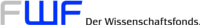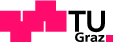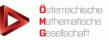# Fall School: Discrete Geometry and Topology

TU Graz, September 28 — October 6, 2016
 Go to: Home — Organization — Lecturers — Abstracts — Schedule

## Abstracts of courses

### Herbert Edelsbrunner: Computational Geometry and Topology

•  Delaunay Complexes. The Delaunay triangulation and its dual Voronoi diagram are among the most fundamental concepts in computational geometry. After defining them, we present some of their pertinent properties, and we discuss their generalization to weighted point data.
•  Discrete Morse Theory. The radius function on the Delaunay triangulation fits a recent generalization of Forman's discrete Morse theory. We describe this algebraic framework and apply it to elucidate important properties of the Delaunay triangulation.
•  Persistent Homology. Among the many faces of this extension of the classical theory of homology groups is the connection to discrete Morse theory, which it extends by pairing not necessarily incident simplices and derive meaning from this pairing.

### Dan Halperin: The Arts and Crafts of Minkowski Sums

The Minkowski sum of two sets P and Q in Euclidean space is the result of adding every point (position vector) in P to every point in Q. Minkowski sums constitute an essential tool in geometric computing, used in a variety of domains including robotics, solid modeling, 3D printing and many more. At the same time they are an inexhaustible source of intriguing mathematical and computational problems. The course starts by walking through the basics of Minkowski sums - structure and complexity - in two and three dimensions. We then review fundamental issues and prevalent practices in computing Minkowski sums. Finally, we describe how Minkowski sums are used to solve problems in an array of applications.

### Tim Hoffmann: Discrete curvatures and discrete integrable systems

This course looks into curvature in discrete differential geometry. Curvature is a central notion in classical differential goemetry of curves and surfaces. Likewise, discrete notions of curvature are present in many theories in discrete differential geometry. We will discuss concepts for curvature that are motivated by and harmonize with the integrable structure found in many of these theories. Starting form curvatures for discrete curves we aim to see how some of them emerge naturally form integrable deformations. These concepts can then be applied to introduce curvatures for quadrilateral meshes in space, giving rise to notions of discrete surfaces of constant curvature that reproduce the integrable structure of their smooth counterparts.

### Helmut Pottmann: Discretization and Optimization in Applied Geometry

This course presents tools from discrete and computational differential geometry and shows how to use them within appropriate optimization strategies to solve a variety of problems in Applied Geometry. The applications in particular include digital fabrication and freeform architecture. We discuss the modeling of polyhedral meshes and developable surfaces, due to their importance in various fabrication technologies, and we study how practical requirements in the construction of architectural freeform structures motivate the development of new concepts in discrete differential geometry and how geometry is providing important support in the realization of challenging projects in contemporary architecture.

[Slides]

### Raman Sanyal: A sampler of geometric combinatorics

Polytopes and polyhedra are at the heart of discrete geometry. Many of their geometric properties can be understood with the help of combinatorics. In this course we will explore what the geometry of polytopes can do for combinatorics. To this end we will see how combinatorial objects such as posets, trees, colorings and so on are modelled, counted, and manipulated through polytopes.

### Emo Welzl: Crossing-free Geometric Graphs

Abstract: We take a tour through several questions in algorithmic and extremal counting of combinatorial objects, with emphasis on how they connect to each other in their solutions. Among these problems are (i) counting of crossing-free geometric graphs of several types on so-called wheel-sets (point sets in the plane with all but one point extremal), (ii) investigating the minimal number of crossings a drawing of the complete graph with straight line edges in the plane must have, (iii) counting the number of simplices spanned by d+1 points in a finite set of n points in d-space (simplicial depth), and (iv) counting the number of facets of the convex hull of n points in d-space (in particular, if d is close to n).